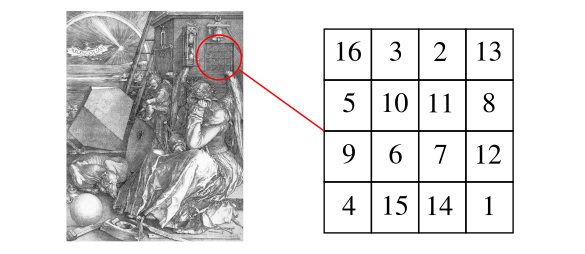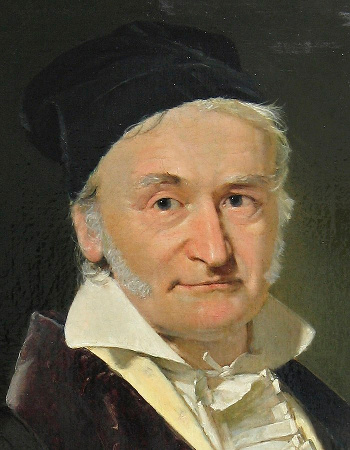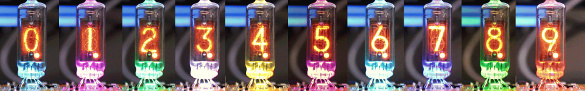### Crazy Number Sequences

January 28, 2019

Mathematics may have helped to land men on the Moon and robots on Mars, but mathematicians do not always keep their pencils to the grindstone. They agree with the "all work, no play" adage and mix a lot of play into their mathematics. An early historical example of such recreational mathematics would be magic squares, traced back more than two thousand years but surprisingly never discovered by the Greek mathematicians.Melencolia I, a 1514 engraving by Albrecht Dürer (1471–1528), now located at the Staatliche Kunsthalle Karlsruhe. This engraving contains a 4x4 magic square based on the number 34. The sum 34 is found in the rows, columns, diagonals, but also in each of the quadrants and the center four squares, among other places. (Via Wikimedia Commons.)

Palindromes are words or sentences that read the same forwards and backwards. A good technological example of a palindrome is the word, radar. The Palindrome principle can also be applied to numbers to create palindromic numbers. These numbers are sequence A002113 in the On-Line Encyclopedia of Integer Sequences (OEIS), and a few examples just below the number one million (which is obviously not a palindrome) are 990099, 991199, 992299, 993399, 994499, 995599, 996699, 997799, 998899, and 999999; and f303f, f313f, f323f, f333f, f343f, f353f, f363f, f373f, f383f, f393f, f3a3f, f3b3f, f3c3f, f3d3f, f3e3f, f3f3f, f404f, and f414f for hexadecimal numbers These are very easily generated in a high-level language, and my PHP source code for such a program can be found here.

Champernowne's constant is the apparently useless number, 0.12345678910111213141516..., which is sequence A033307 in the OEIS. It's construction is obvious, and the concept can be applied to other number bases as well, such as base-2 (binary), 0.11011100101110111... Seeing the sequence of natural numbers contained in Champernowne's constant brings to mind an interesting anecdote from the history of mathematics.

Early in their lives, even famous mathematicians couldn't add two-plus-two; but, unlike ordinary people, after learning that this sum was four, they quickly progressed through higher levels of mathematical abstraction. Carl Friedrich Gauss (1777-1855) was one such mathematician who also excelled as a physicist. As the story goes, Gauss was an eight year old child among other elementary school students who were given a lengthy mathematics problem by their teacher. This is a common tactic of teachers who need time to grade homework or tests.

The assignment was to add together all the numbers from one to a hundred. Gauss immediately arrived at the correct answer, 5050, with no written calculation. He realized that you could pair most of the numbers, 1 with 99, 2 with 98, etc., to give you 49 sums of a hundred each. Adding the additional 100 and 50 immediately gave the answer, 5050. There's a general formula for calculating the sum of such a series up to the number n,This gives us Gauss' answer when n = 100.Carl Friedrich Gauss (1777-1855).

Gauss has a plethora of things named after him, including a unit of magnetism, the gauss, that's been superseded by the tesla.

As I wrote in a recent article (Great Circle Routes, June 25, 2018), Gauss did an experiment in the 1820s to determine whether space was curved; that is, non-Euclidean.

He used his era's version of a laser theodolite, called a heliotrope, to survey a triangle between three mountains - Brocken, Hohenhagen, and Inselberg.

This triangle had sides of length 69, 85 and 107 kilometers, but even such a large triangle has the now known difference in the sum of angles from 180 degrees of just 0.1 picoradians.

(An 1840 oil portrait by the danish painter Christian Albrecht Jensen (1792-1870), via Wikimedia Commons)

If we write the decimal digits 1-9 in sequence and insert a random addition or multiplication operation between digits, as in 1 + 2 x 3 + 4 x 5 x 6 x 7 + 8 x 9, what range of numbers would we get? We need to remember the the order of operations in which we always multiply before we add. In the given example, we get 919. Since there are just eight operations, we can do an exhaustive search of all 256 different possibilities. A simple PHP program (found here) gives us the following as the minimum and maximum results:
1 x 2 + 3 + 4 + 5 + 6 + 7 + 8 + 9 = 44

1 + 2 x 3 x 4 x 5 x 6 x 7 x 8 x 9 = 362881
This serves as an introduction to a lengthy 2014 paper on arXiv by Inder J. Taneja, who was formerly a professor of mathematics at the Universidade Federal de Santa Catarina (Florianópolis, Brazil). Taneja considers both ascending and descending decimal digits, parenthetical grouping, and also adds exponentiation to the mathematical operator mix. Also allowed are numbers formed by the concatenation of successive digits, as the following examples show.
346 = 12345 + 6 x 7 x 8 + 9

346 = 9 x 8 + 7 + 65 x 4 + 3 x 2 + 1

514 = 1 + 23 x 4 + 56 x 7 + 89

514 = 98 + 76 x 5 + 4 + 32 x 1

6096 = 12 x 3 x 45 + 6 x 7 x 8 x 9

6096 = 9 + 87 x 65 + 432 x 1

9261 = 123 x 4 + 5 x 6 x 78 + 9

9261 = (9 + 8 + 76 + 54) x 3 x 21
By adding exponentiation, we can create numbers less than 44, as the example, 1 = 123456789, shows. However, there are numbers that can't be generated in either an increasing or decreasing digit sequence, one small number example being 52. One such larger number is 11108. Timothy Wylie of the University of Texas - Rio Grande Valley (Edinburg, Texas) has published a more recent paper on arXiv that examines this idea when applied to number bases other than ten.Old school numerical display - An array of IN16 Nixie tubes. The instruments in my early laboratories contained mostly analog voltmeters, often with a mirrored strip to allow an accurate reading of the indicator position without parallax. The first digital instruments had displays using these Nixie tubes. (Created from a Wikimedia Commons image by "FxDev.")

### References:

Linked Keywords: Mathematics; Apollo 11; land men on the Moon; Curiosity (rover); robots on Mars; mathematician; pencil; grindstone; All work and no play makes Jack a dull boy; "all work, no play" adage; history; historical; recreational mathematics; magic square; ancient Greece; Greek; Melencolia I; engraving; Albrecht Dürer (1471–1528); Staatliche Kunsthalle Karlsruhe; palindrome; technology; technological; radar; natural number; palindromic number; sequence A002113; On-Line Encyclopedia of Integer Sequences (OEIS); hexadecimal; high-level language; PHP; source code; palindrome.php; Champernowne's constant; sequence A033307; radix; number base; ninary number; base-2; anecdote; history of mathematics; mathematical abstraction; Carl Friedrich Gauss (1777-1855); physicist; child; elementary school; student; mathematical problem; mathematics problem; teacher; tactic (method); grading in education; homework; test (assessment); calculation; formula; plethora; things named after Carl Friedrich Gauss; unit of measurement; magnetism; gauss; tesla; experiment; spacetime; curvature of space; Euclidean space; non-Euclidean; laser; theodolite; heliotrope (instrument); surveying; survey; triangle; mountain; Brocken; Hoher Hagen (Dransfeld); Hohenhagen; Großer Inselsberg; kilometer; angle; picoradian; Christian Albrecht Jensen (1792-1870); Wikimedia Commons; decimal digit; sequence; randomness; random; addition; multiplication; operation (mathematics); order of operations; crazy seq.php; arXiv; Inder J. Taneja; professor; Universidade Federal de Santa Catarina (Florianópolis, Brazil); bracket; parenthetical grouping; exponentiation; concatenation; Timothy Wylie; University of Texas - Rio Grande Valley (Edinburg, Texas); old school; numerical digit; display; Nixie tube; scientific instrument; laboratory; galvanometer; analog voltmeter; mirror; parallax; digital instrument.